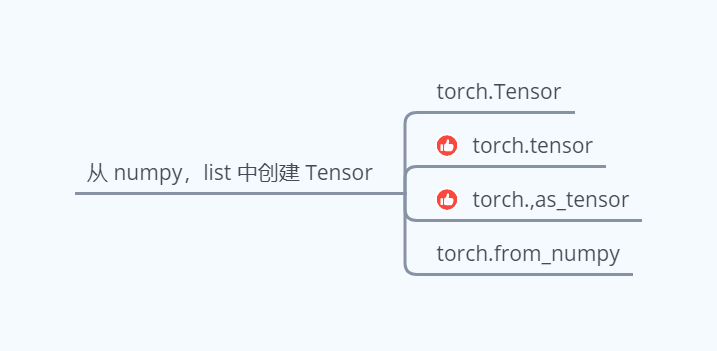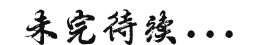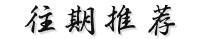• 功能：完成数组numpy到tensor的转换。 示例代码： import torch import numpy as np a = np.ones(5) b = torch.from_numpy(a) print(type(a)) print(type(b)) 输出结果： <class 'numpy.ndarray'> <class...
功能：完成数组numpy到tensor的转换。
示例代码：
import torch
import numpy as np

a = np.ones(5)
b = torch.from_numpy(a)
print(type(a))
print(type(b))

输出结果：
<class 'numpy.ndarray'>
<class 'torch.Tensor'>

参考链接：
https://blog.csdn.net/qq_33345917/article/details/86552152


展开全文• 从Tensor到numpy tensor = torch.Tensor([0,1]) # 如果参数不是一个list，而是一个int，将会返回该int长的内容未定义的Tensor ...从numpy到Tensor array = np.random.rand(3) torch.from_numpy(array) ...
从Tensor到numpy
tensor = torch.Tensor([0,1]) # 如果参数不是一个list，而是一个int，将会返回该int长的内容未定义的Tensor
tensor_numpy = tensor.numpy()

从numpy到Tensor
array = np.random.rand(3)
torch.from_numpy(array)



展开全文• 关注"AI机器学习与深度学习算法"公众号选择"星标"公众号，原创干货，第一时间送达从数组、列表对象创建Numpy ...Array 数组或者 List 列表容器，再转换 Tensor 类型。(为了方便描述，后面将 Numpy Array 数组称为...关注"AI机器学习与深度学习算法"公众号选择"星标"公众号，原创干货，第一时间送达从数组、列表对象创建Numpy Array 数组和 Python List 列表是 Python 程序中间非常重要的数据载体容器，很多数据都是通过 Python 语言将数据加载至 Array 数组或者 List 列表容器，再转换到 Tensor 类型。(为了方便描述，后面将 Numpy Array 数组称为数组，将 Python List 列表称为列表。)PyTorch 从数组或者列表对象中创建 Tensor 有四种方式：torch.Tensortorch.tensortorch.as_tensortorch.from_numpy>>> import torch>>> import numpy as np>>> array = np.array([1, 2, 3])>>> list = [4, 5, 6]# 方式一：使用torch.Tensor类>>> tensor_array_a = torch.Tensor(array)>>> tensor_list_a = torch.Tensor(list)>>> print(isinstance(tensor_array_a, torch.Tensor)      , tensor_array_a.type())True torch.FloatTensor>>> print(isinstance(tensor_list_a, torch.Tensor)      , tensor_list_a.type())True torch.FloatTensor# 方式二：使用torch.tensor函数>>> tensor_array_b = torch.tensor(array)>>> tensor_list_b = torch.tensor(list)>>> print(isinstance(tensor_array_b, torch.Tensor)      , tensor_array_b.type())True torch.LongTensor>>> print(isinstance(tensor_list_b, torch.Tensor)      , tensor_list_b.type())True torch.LongTensor# 方式三：使用torch.as_tensor函数>>> tensor_array_c = torch.as_tensor(array)>>> tensor_list_c = torch.as_tensor(list)>>> print(isinstance(tensor_array_c, torch.Tensor)      , tensor_array_c.type())True torch.LongTensor>>> print(isinstance(tensor_list_c, torch.Tensor)      , tensor_list_c.type())True torch.LongTensor# 方式四：使用torch.from_numpy函数>>> tensor_array_d = torch.from_numpy(array)# tensor_list_d = torch.from_numpy(list) error code>>> print(isinstance(tensor_array_d, torch.Tensor)      , tensor_array_d.type())True torch.LongTensor# print(isinstance(tensor_list_d, torch.Tensor)#       , tensor_list_d.type())通过上面代码的执行结果可以简单归纳出四种创建 Tensor 方式的差异：只有 torch.Tensor 是类，其余的三种方式都是函数；torch.Tensor、torch.tensor 和 torch.as_tensor 三种方式可以将数组和列表转换为 Tensor，但是 torch.from_numpy 只能将数组转换为 Tensor(为 torch.from_numpy 函数传入列表，程序会报错)；从程序的输出结果可以看出，四种方式最终都将数组或列表转换为 Tensor(使用 isinstance 返回的结果都为 True)，「但是转换后的 Tensor 数据类型却有所不同，在上一小节区分 torch.Tensor 和 torch.tensor 的时候提到过，当接收数据内容时，torch.Tensor 创建的 Tensor 会使用默认的全局数据类型，而 torch.tensor 创建的 Tensor 会使用根据传入数据推断出的数据类型。」 可以通过 torch.get_default_dtype() 来获取当前的全局数据类型，也可以通过 torch.set_default_dtype(torch.XXXTensor) 来设置当前环境默认的全局数据类型；>>> import torch>>> import numpy as np>>> array = np.array([1, 2, 3])>>> print(array)int64# 获取当前全局环境的数据类型>>> print(torch.get_default_dtype())torch.float32# 方式一：使用torch.Tensor类>>> tensor_array_a = torch.Tensor(array)>>> print(tensor_array_a.type())torch.FloatTensor# 方式二：使用torch.tensor函数>>> tensor_array_b = torch.tensor(array)>>> print(tensor_array_b.type())torch.LongTensor# 设置当前全局环境的数据类型为torch.DoubleTensor>>> torch.set_default_tensor_type(torch.DoubleTensor)>>> tensor_array_a = torch.Tensor(array)>>> print(tensor_array_a.type())torch.DoubleTensor>>> tensor_array_b = torch.tensor(array)>>> print(tensor_array_b.type())torch.LongTensor「PyTorch 默认的全局数据类型为 torch.float32，因此使用 torch.Tensor 类创建 Tensor 的数据类型和默认的全局数据类型一致，为 torch.FloatTensor，而使用 torch.tensor 函数创建的 Tensor 会根据传入的数组和列表中元素的数据类型进行推断，此时 np.array([1, 2, 3]) 数组的数据类型为 int64，因此使用 torch.tensor 函数创建的 Tensor 的数据类型为 torch.LongTensor。」 使用 torch.set_default_tensor_type(torch.DoubleTensor) 更改了默认的全局数据类型之后，使用 torch.Tensor 生成的 Tensor 数据类型会变成更改后的数据类型，而使用 torch.tensor 函数生成的 Tensor 数据类型依然没有改变，「当然可以在使用 torch.tensor 函数创建 Tensor 的时候指定 dtype 参数来生成指定类型的 Tensor。」PyTorch 提供了这么多方式从数组和列表中创建 Tensor。一般来说，不推荐使用 torch.Tensor 类，因为不仅可以为 torch.Tensor 传入数据还可以传入形状(torch.tensor 只能传入数据，这样单一的功能可以防止出错)，当为 torch.Tensor 传入形状时会生成指定形状且包含未初始化数据的 Tensor，如果忘记替换掉这些未初始化的值，直接输入到神经网络中，可能会让神经网络输出 NAN 或者 INF。「如果不考虑性能的话，推荐使用 torch.tensor。如果考虑性能，推荐使用 torch.as_tensor(torch.from_numpy 只能接受数组类型)，因为使用 torch.as_tensor 生成的 tensor 会和数组共享内存，从而节省内存的开销。」Tips：增添了自己的理解与看法 龙良曲深度学习与PyTorch入门实战：https://study.163.com/course/introduction/1208894818.htm【PyTorch入门笔记】简单回归案例【PyTorch入门笔记】简单回归案例实战【PyTorch入门笔记】手写数字问题【PyTorch入门笔记】基本数据类型【机器学习入门笔记】11-9 SVM思想解决回归问题【机器学习入门笔记】什么是决策树？展开全文• tensorflow tensor或numpy数组打印出全部值 1、加入以下代码 import numpy as np np.set_printoptions(threshold=np.inf) parser = argparse.ArgumentParser() #如果提示parser找不的错误，可以把这一行注释掉 ...

tensorflow tensor或numpy数组打印出全部值
1、加入以下代码
import numpy as np
np.set_printoptions(threshold=np.inf)
parser = argparse.ArgumentParser()    #如果提示parser找不到的错误，可以把这一行注释掉



展开全文• 构造张量数组： 最简单的方式： tensor_list=[tensor1,tensor2] 常用的方式(这个方式可以用于for循环） ...批量处理张量数组里面的张量，之后将其存储一个新的张量数组中 new_tensor_l...
• 它可以是一个数（标量），一维数组（向量），二维数组或更高维数组Tensor支持GPU加速。 创建Tensor 几种常见创建Tensor的方法 举例： 生成全为1的Tensor 生成全为0的Tensor 随机生成 对角线元素...机器学习 Pytorch 深度学习
• 关注"AI机器学习与深度学习算法"公众号选择"星标"公众号，原创干货，第一时间送达从数组、列表对象创建Numpy ...Array 数组或者 List 列表容器，再转换 Tensor 类型。(为了方便描述，后面将 Numpy Array 数组称为...
• 我主要在数据前期预处理的情况下遇到这个问题，大概就是想把excel表格中的label和feature都提取出来转换成tensor，但在label的numpy数组转换成tensor出现以下错误 分析错误 TypeError: Expected binary or ...
• tensorflow里面的张量也就是tensor其实就是numpy里面的ndarry，也就是多维数组，一维数组是个列表，二维数组是个矩阵，当我们的维度上升了三维四维甚至更高维度的时候，它就是一个张量，同学们不要被这个单词给吓...
• 我的需求是有一个15*4的tensor, 有一个15*1的index vector, 希望从tensor里面拿对应的15*1的vector 查了半天gather未果, 因为gather()只能拿一个slice gather_nd也不是很好的解决方法, 不过可以实现, 需要把...
• 在用pytorch训练神经网络时，我们...一、numpy到tensor 首先我们要引入必要的包： import numpy as np import torch 然后创建一个numpy类型的数组： x = np.ones(5) print(type(x)) 这里创建了一个一维...python pytorch numpy
• 主要学习torchvision.transforms，包括常用的图像操作，例如：随机切割，旋转，数据类型转换，图像到tensor ,numpy 数组到tensor , tensor 到 图像等。 transforms.Compose可以组合几个变换连续一起操作 比如 ...深度学习
• 可以将Tensor当作一个数据结构，里面的数据可以是一个数，也可以是1维数组，2维的矩阵，以及更高维的矩阵。 TensorflowJS支持CPU和GPU两种后端。虽然输入的数据都是Tensor，但是对数据的处理有较大的差异。如果是CPU...
• Tensor，又名张量，它不仅在PyTorch中出现过，它也是Theano、TensorFlow、 Torch和MxNet中重要的数据结构。关于张量的本质不乏深度的剖析，但从工程角度来讲，可简单地认为它就是一个数组，且支持高效的科学计算。它...
• 功能：完成数组numpy到tensor的转换。 栗子： import torch import numpy as np a = np.ones(5) b = torch.from_numpy(a) print(type(a)) print(type(b)) 输出结果： <class 'numpy.ndarray'> <...
• 例如用法跳转 tensor模块在嵌入式应用中的使用 看到这个 tensor模块的用法示例 创建多维张量： // Create an array for storing bitmaps with variadic constructor int constexpr width = 1024 ; int constexpr ...
• 从Numpy 到Tensor .from_numpy()方法 把numpy数据转换成张量 import torch import numpy as np a = np.array([2,3.3]) # 生成一个一维数组 并指定初值 print("a 的值是：{}".format(a)) a = torch.from_numpy(a) #把...numpy 人工智能 python 深度学习
• 希望大家直接上面的网址去查看...Tensor可以是一个数（标量）、一维数组（向量）、二维数组（矩阵）或更高维的数组（高阶数据） Tensor和numpy的ndarrays类似，不同在于pytorch的tensor支持GPU加速 导包： ...
• pytorch入门之Tensor tensor是什么 Tensor译为张量。我的理解Tensor和多维数组比较...在pytorch中，tensor可以和ndarray（numpy中）相互转换，而且pytorch中的tensor可以方便的放GPU上进行计算。 使用tensor pytor...pytorch
• ## TensorFlow中tensor

千次阅读 2020-04-28 05:56:43
N维数组(N维张量)表征了数据的不同形状，比如0维张量表示标量、1维张量表示向量，2维张量表示矩阵，下面我们直观的给出0维4维的张量，更高维度的张量很少用，而且理解起来也比较困难，这里...tensorflow 神经网络 深度学习
• 一、名词解释 在Tensorflow里： ...说白了就是多维数组。 标量是张量（实数值映射实数值） 向量是张量 矩阵是张量 矩阵的矩阵是张量 所以在tensorflow 中： constant 常量张量 variable 变量，一般是
• 因为 PyTorch 是一个使用 Python语言的神经网络库, Torch 很好用, 但是 Lua 又不是特别流行, 所有开发团队将 Lua 的 Torch 移植了更流行的语言 Python 上. Torch 自称为神经网络界的 Numpy, 因为他能将 torch ...code
• 数组、列表对象创建Numpy Array 数组和 Python List 列表是 Python 程序中间非常重要的数据载体容器，很多数据都是通过 Python 语言将数据加载至 Array 数组或者 List 列表容器，再转换 Tensor 类型。...
• 使用pytorch获取tensor每行中...最近刚刚入门pytorch，测试完模型计算准确率需要R@1，R@5等，想获取一下tensor每行前k个大的数，奈何搜了一圈就没说点子上的…参照numpy数组，使用了一下tensor.argsort(),成功。 ...pytorch
• CPU tensor转GPU tensor： cpu_imgs.cuda() GPU tensor 转CPU tensor： gpu_imgs.cpu() ...note：GPU tensor不能直接转为numpy数组，必须先转CPU tensor。 _, pred_int = torch.max(outputs.dat.深度学习
• 1. CPU tensor转GPU tensor： cpu_imgs.cuda() 2. GPU tensor 转CPU tensor： ...5.note：GPU tensor不能直接转为numpy数组，必须先转CPU tensor。 6. 如果tensor是标量的话，可以直接使用.
• ## Pytorch（一）入门：Tensor基础

万次阅读 多人点赞 2018-09-21 15:23:25
在numpy中，我们是通过shape来获取数组的形状，而在我们的tensor当中，我们使用size来得到形状。 有时候，我们需要对数组形状进行改变，我们可以采用 .view() 的方式 可以注意test5通过test4变形的时候，是...pytorch入门
• 一般来说，需要将tensor转变为numpy类型的数组从而保存图片，这样的过程比较繁琐，Pytorch提供了save_image()函数，可直接将tensor保存为图片，若tensor在cuda上也会移CPU中进行保存。 ) 参数： tensor (Tensor ...深度学习 python
• 问题详情：在获得模型结果output后希望转化为numpy的数组类型，但是无法直接使用numpy()，需要先将数据从GPU中取CPU中 解决方法：先调用cpu()再调用numpy()，比如 predict_train.cpu().numpy() ...python pytorch...